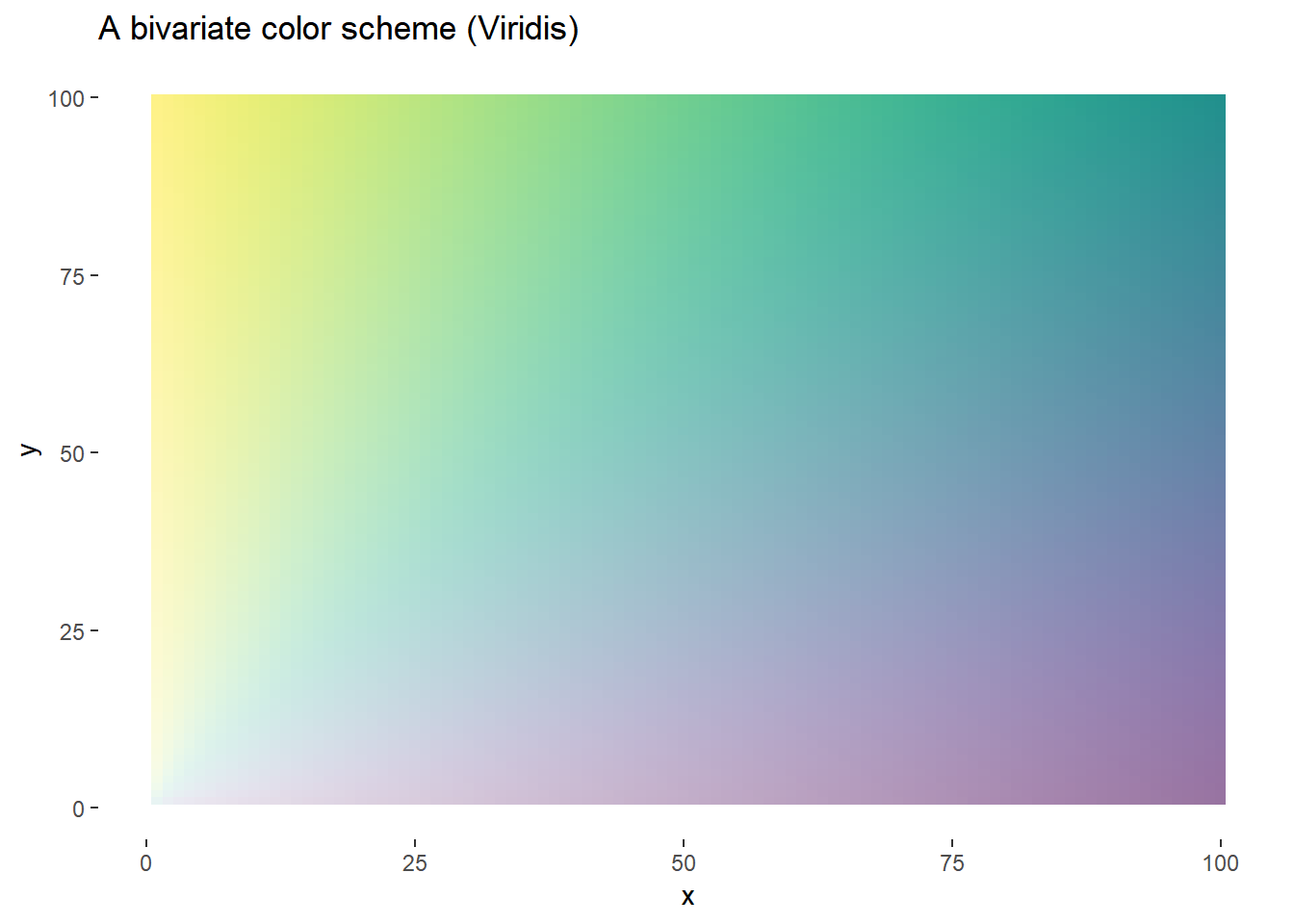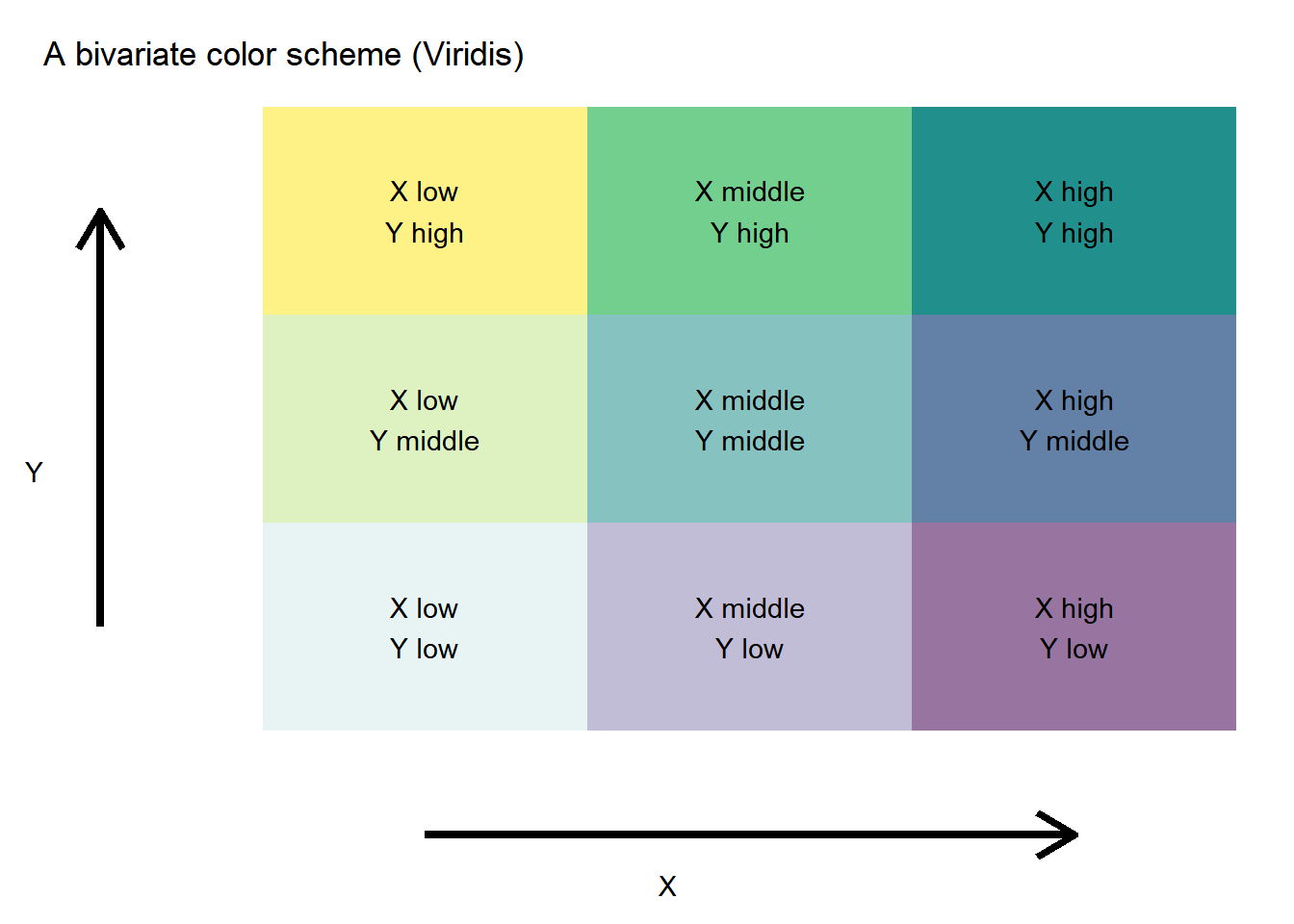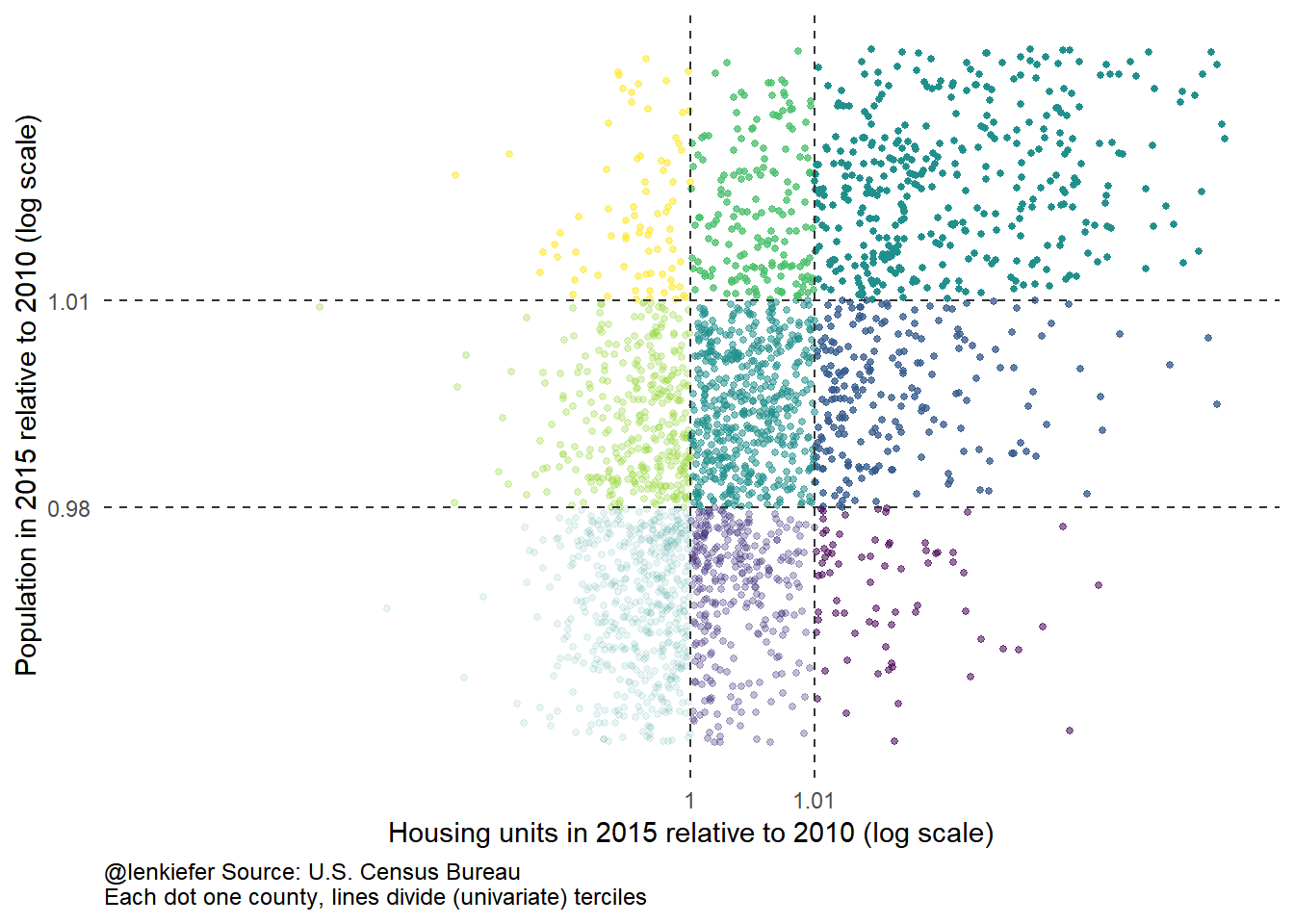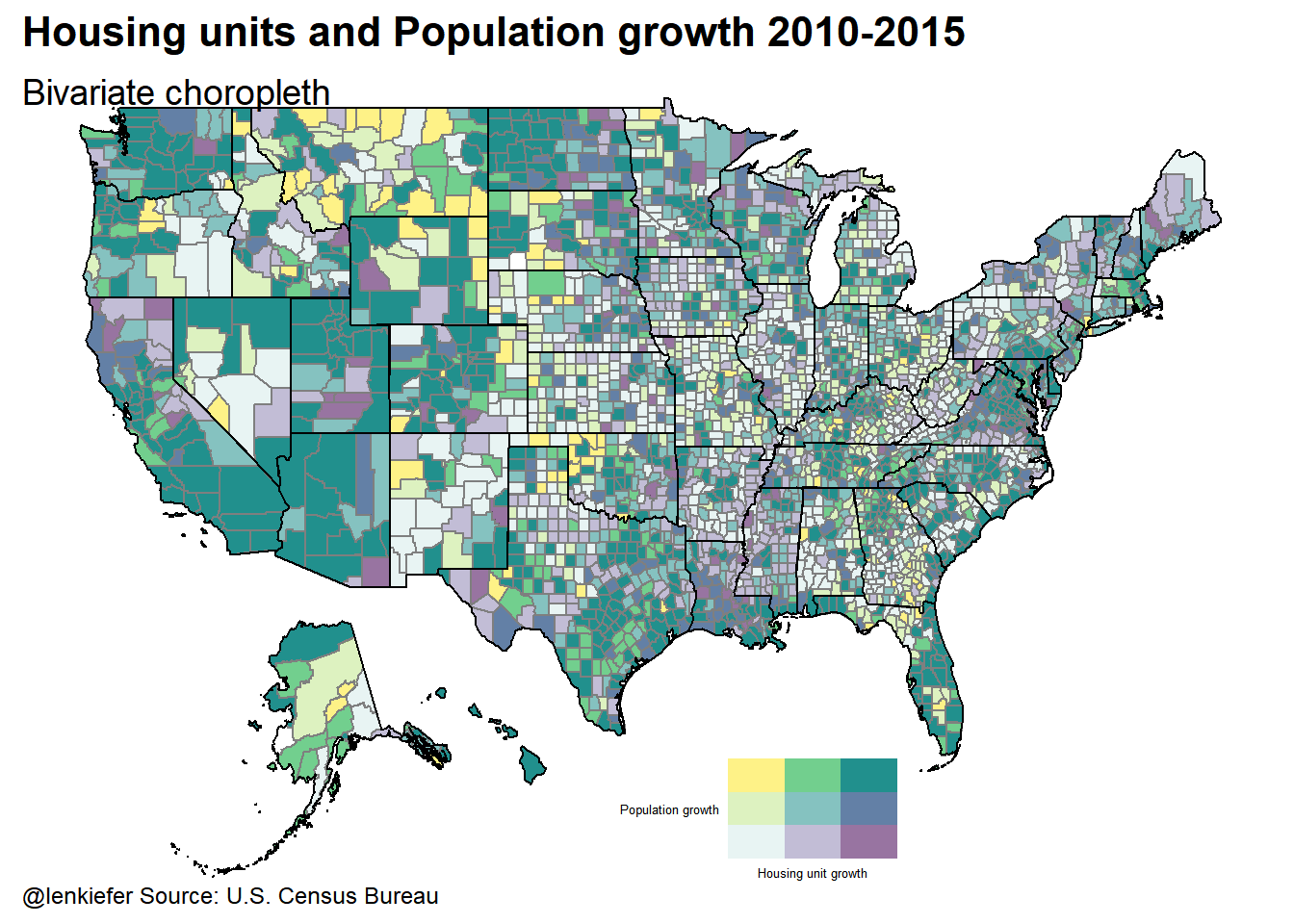# Bivariate choropleth maps with R

NOTE: After I posted this (like within 5 minutes) I found this post which also constructs bivariate chropleths in R.

IN THIS POST I WANT TO REVISIT SOME MAPS I MADE LAST YEAR. At that time, I was using Tableau to create choropleth maps, but in this post I want to reimagine the maps and make them in R.

Last year in this post we looked at the relationship between population growth and the growth in housing units from 2010 to 2015. While the Census has released national level estimates for population, the county level estimates are not updated yet. However, you can get estimates for 2010 through 2015, which is what I used last year and will use again this year.

# Bivariate choropleth

In last year’s post I wanted to compare population growth to expansion of the housing supply. I constructed a ratio of the growth in population from 2010 to 2015 to the growth in housing units from 2010 to 2015 by county. Then I plotted this ratio on a diverging color scheme.

Instead, we could use a bivariate color scale. If you are into reading, see an extended discussion here.

Or you could do like me. Just jump in we’ll and figure it out as we go. Here goes!

``library(tidyverse)``
``## Warning: package 'tidyverse' was built under R version 3.5.1``
``## -- Attaching packages -------------------------------------------------------------------------------------------------------------------------------------------------------------------------------- tidyverse 1.2.1 --``
``````## v ggplot2 3.0.0     v purrr   0.2.5
## v tibble  1.4.2     v dplyr   0.7.6
## v tidyr   0.8.0     v stringr 1.3.1
## v readr   1.1.1     v forcats 0.3.0``````
``## Warning: package 'purrr' was built under R version 3.5.1``
``## Warning: package 'stringr' was built under R version 3.5.1``
``````## -- Conflicts ----------------------------------------------------------------------------------------------------------------------------------------------------------------------------------- tidyverse_conflicts() --
``library(viridis)``
``## Loading required package: viridisLite``
``````library(readxl)
library(stringr)
d<-expand.grid(x=1:100,y=1:100)

ggplot(d, aes(x,y,fill=atan(y/x),alpha=x+y))+
geom_tile()+
scale_fill_viridis()+
theme(legend.position="none",
panel.background=element_blank())+
labs(title="A bivariate color scheme (Viridis)")``````We could allow this scheme to vary smoothly, or it might be easier to see what’s going on, but using a discrete scale. A 3x3 grid seems to work pretty well.

``````# Add some labels
d<-expand.grid(x=1:3,y=1:3)
#dlabel<-data.frame(x=1:3,xlabel=c("X low", "X middle","X High"))
d<-merge(d,data.frame(x=1:3,xlabel=c("X low", "X middle","X high")),by="x")
d<-merge(d,data.frame(y=1:3,ylabel=c("Y low", "Y middle","Y high")),by="y")

g.legend<-
ggplot(d, aes(x,y,fill=atan(y/x),alpha=x+y,label=paste0(xlabel,"\n",ylabel)))+
geom_tile()+
geom_text(alpha=1)+
scale_fill_viridis()+
theme_void()+
theme(legend.position="none",
panel.background=element_blank(),
plot.margin=margin(t=10,b=10,l=10))+
labs(title="A bivariate color scheme (Viridis)",x="X",y="Y")+
theme(axis.title=element_text(color="black"))+
# Draw some arrows:
geom_segment(aes(x=1, xend = 3 , y=0, yend = 0), size=1.5,
arrow = arrow(length = unit(0.6,"cm"))) +
geom_segment(aes(x=0, xend = 0 , y=1, yend = 3), size=1.5,
arrow = arrow(length = unit(0.6,"cm")))
g.legend``````### Get some data

I happened to have the data in a spreadsheet called pophous.xlsx. You can get estimates direct from the U.S. Cesnsus Bureau (here for housing units and here for population).

I rearranged my data in a spreadsheet like this:This was of course, before I wised up about readxl. But since I had these data around, we’ll just start here.

``````# Load data and check

df<-left_join(dfh,dfp,by="fips")
df <- df %>% select(-h2010apr,-h2010base,-p2010apr,-p2010base)

map_if(is_numeric,round,0) %>%
data.frame() %>% as.tbl())))``````
``````## Warning: Deprecated

## Warning: Deprecated

## Warning: Deprecated

## Warning: Deprecated

## Warning: Deprecated

## Warning: Deprecated

## Warning: Deprecated

## Warning: Deprecated

## Warning: Deprecated

## Warning: Deprecated

## Warning: Deprecated

## Warning: Deprecated

## Warning: Deprecated``````
fips h2010 h2011 h2012 h2013 h2014 h2015 p2010 p2011 p2012 p2013 p2014 p2015
1 1001 22152 22284 22352 22670 22751 22847 54660 55253 55175 55038 55290 55347
2 1003 104248 104701 105264 106227 107368 108564 183193 186659 190396 195126 199713 203709
3 1005 11827 11808 11841 11816 11799 11789 27341 27226 27159 26973 26815 26489
4 1007 8982 8972 8972 8972 8977 8986 22861 22733 22642 22512 22549 22583
5 1009 23887 23875 23862 23841 23826 23817 57373 57711 57776 57734 57658 57673
6 1011 4492 4483 4477 4468 4461 4456 10887 10629 10606 10628 10829 10696

After clearning our data are arranged with state/county FIPS numbers and then variables p2010-p2015 and h2010-h2015 corresponding to population estimates for each year 2010-2015 and housing units for each year 2010-2015 respectively.

The FIPS numbers are stored as numbers (thanks Excel!) and we’d like to rearrange the data, so let’s do some tidying.

``````# gather up columns by fips
df2<-df %>% gather(var,value,-fips)

# Create a type varialbe (h for housing, p for population) & year variable
df2<-df2 %>% mutate( type=substr(var,1,1), year=as.numeric(substr(var,2,5)))

# spread back out housing units and population
df3 <- df2 %>% select(fips,type,year,value) %>% spread(type,value)

# turn fips into string by padding with a 0

# comput housing units and population in 2010
df3.sums<-df3 %>% group_by(fips) %>%  summarize(h2010= h[sum(year==2010)],
p2010= p[sum(year==2010)])
df3<-left_join(df3,df3.sums,by="fips")

# create variables for housing unites (hr) and population (pr) relative to 2010
# also create ratio
df3 <- df3 %>% mutate(hr=h/h2010, pr=p/p2010, ratio=(h/h2010)/(p/p2010))

# Check it out
map_if(is_numeric,round,0) %>%
data.frame() %>% as.tbl())))``````
``````## Warning: Deprecated

## Warning: Deprecated

## Warning: Deprecated

## Warning: Deprecated

## Warning: Deprecated

## Warning: Deprecated

## Warning: Deprecated

## Warning: Deprecated

## Warning: Deprecated``````
fips year h p h2010 p2010 hr pr ratio
1 01001 2010 22152 54660 22152 54660 1 1 1
2 01001 2011 22284 55253 22152 54660 1 1 1
3 01001 2012 22352 55175 22152 54660 1 1 1
4 01001 2013 22670 55038 22152 54660 1 1 1
5 01001 2014 22751 55290 22152 54660 1 1 1
6 01001 2015 22847 55347 22152 54660 1 1 1

Now things are looking a lot better.

Let’s filter to just 2015 and get ready to make our map.

First, for housing units and population (both relative to 2010) we’ll compute terciles (divide the range into 3 parts each with 1/3 of observations). We’ll then categorize each observation as falling within one of these terciles. Then, we’ll let these terciles be ordered 1 through 3 and construct a scatterplot.

``````d2015<-filter(df3,year==2015)

# compute quantiles (dividing into 3rds)
h.v<-quantile(d2015\$hr,c(0.33,0.66,1))
p.v<-quantile(d2015\$pr,c(0.33,0.66,1))

d2015<- d2015 %>% mutate(y= ifelse(pr<p.v,1,ifelse(pr<p.v,2,3)) ,
x= ifelse(hr<h.v,1,ifelse(hr<h.v,2,3))  )

ggplot(data=d2015,aes(x=hr,y=pr,color=atan(y/x),alpha=x+y))+
geom_point(size=1)+  guides(alpha=F,color=F)+
geom_hline(yintercept=p.v,color="gray20",linetype=2)+
geom_vline(xintercept=h.v,color="gray20",linetype=2)+
scale_color_viridis(name="Color scale")+theme_minimal()+
theme(plot.caption=element_text(size = 9, hjust=0),
panel.grid=element_blank()) +

labs(x="Housing units in 2015 relative to 2010 (log scale)",
y="Population in 2015 relative to 2010 (log scale)",
caption="@lenkiefer Source: U.S. Census Bureau\nEach dot one county, lines divide (univariate) terciles")+
# limit the rang e
scale_x_log10(limits=c(0.95,1.05), breaks=c(h.v),
labels=round(c(h.v),2)) +
scale_y_log10(limits=c(0.95,1.05),breaks=c(p.v),
labels=round(c(p.v),2)) ``````
``## Warning: Removed 646 rows containing missing values (geom_point).``
``## Warning: Removed 1 rows containing missing values (geom_hline).``
``## Warning: Removed 1 rows containing missing values (geom_vline).``Now that we’ve divided up the observations, let’s map them!

``````#################################################################################
### Map Libraries
library(ggthemes)
library(ggalt)
library(maps)``````
``````##
## Attaching package: 'maps'``````
``````## The following object is masked from 'package:purrr':
##
##     map``````
``library(rgeos)``
``````## rgeos version: 0.3-28, (SVN revision 572)
##  GEOS runtime version: 3.6.1-CAPI-1.10.1 r0
##  Linking to sp version: 1.3-1
##  Polygon checking: TRUE``````
``library(maptools)``
``## Loading required package: sp``
``## Checking rgeos availability: TRUE``
``````library(albersusa)
library(grid)
#################################################################################

#################################################################################
states<-usa_composite()  #create a state map thing
smap<-fortify(states,region="fips_state")
counties <- counties_composite()   #create a county map thing
#################################################################################
#add on summary stats by county using FIPS code
counties@data <- left_join(counties@data, d2015, by = "fips")
#################################################################################
cmap <- fortify(counties_composite(), region="fips")
#create state and county FIPS codes
cmap\$state<-substr(cmap\$id,1,2)
cmap\$county<-substr(cmap\$id,3,5)
cmap\$fips<-paste0(cmap\$state,cmap\$county)

#### Make a legend
g.legend<-
ggplot(d, aes(x,y,fill=atan(y/x),alpha=x+y,label=paste0(xlabel,"\n",ylabel)))+
geom_tile()+
scale_fill_viridis()+
theme_void()+
theme(legend.position="none",
axis.title=element_text(size=5),
panel.background=element_blank(),
plot.margin=margin(t=10,b=10,l=10))+
theme(axis.title=element_text(color="black"))+
labs(x="Housing unit growth",
y="Population growth")

gmap<-
ggplot() +
geom_map(data =cmap, map = cmap,
aes(x = long, y = lat, map_id = id),
color = "#2b2b2b", size = 0.05, fill = NA) +
geom_map(data = filter(counties@data,year==2015), map = cmap,
aes(fill =atan(y/x),alpha=x+y, map_id = fips),
color = "gray50") +
#add black state borders (just to see if things are working)
geom_map(data = smap, map = smap,
aes(x = long, y = lat, map_id = id),
color = "black", size = .5, fill = NA) +
theme_map(base_size = 12) +
theme(plot.title=element_text(size = 16, face="bold",margin=margin(b=10))) +
theme(plot.subtitle=element_text(size = 14, margin=margin(b=-20))) +
theme(plot.caption=element_text(size = 9, margin=margin(t=-15),hjust=0)) +
scale_fill_viridis()+guides(alpha=F,fill=F)+
labs(caption="@lenkiefer Source: U.S. Census Bureau",
title="Housing units and Population growth 2010-2015",
subtitle="Bivariate choropleth")

vp<-viewport(width=0.24,height=0.24,x=0.58,y=0.14)

print(gmap)
print(g.legend+labs(title=""),vp=vp)``````### So what?

Well, that was pretty neat.

I think you probably should be careful about interpreting these maps, particularly when you have some counties that might not be estimated as well as others. Still, the ability to display two variables on one map might be handy sometime.

In the past I compared employment and house prices and a bivariate choropleth might be a good option for comparing how these variables evolve. I’ve also go some research going looking at some other aspects of the housing market where such a plot might at least be useful at the data exploration stage.

How could it work for you?